# RD Sharma Solutions For Class 12 Maths Exercise 19.3 Chapter 19 Indefinite Integrals

RD Sharma Solutions for Class 12 Maths Exercise 19.3 Chapter 19 Indefinite Integrals is provided here. The geometrical interpretation of indefinite integral, integration by substitution are the main insights of this exercise. The RD Sharma Solutions Class 12 prepared by experts at BYJU’S can help students understand these concepts clearly, following the latest CBSE patterns.

As a result, students can achieve higher marks in their examinations. To score more in board exams, students can refer to RD Sharma Solutions for Class 12 Maths Chapter 19 Indefinite Integrals Exercise 19.3 from the below links. Some of the essential topics of this exercise are provided below.

• Geometrical interpretation of indefinite integral
• Comparison between differentiation and integration
• Methods of integration
• Integration by substitution

## Download the PDF of RD Sharma Solutions For Class 12 Chapter 19 – Indefinite Integrals Exercise 19.3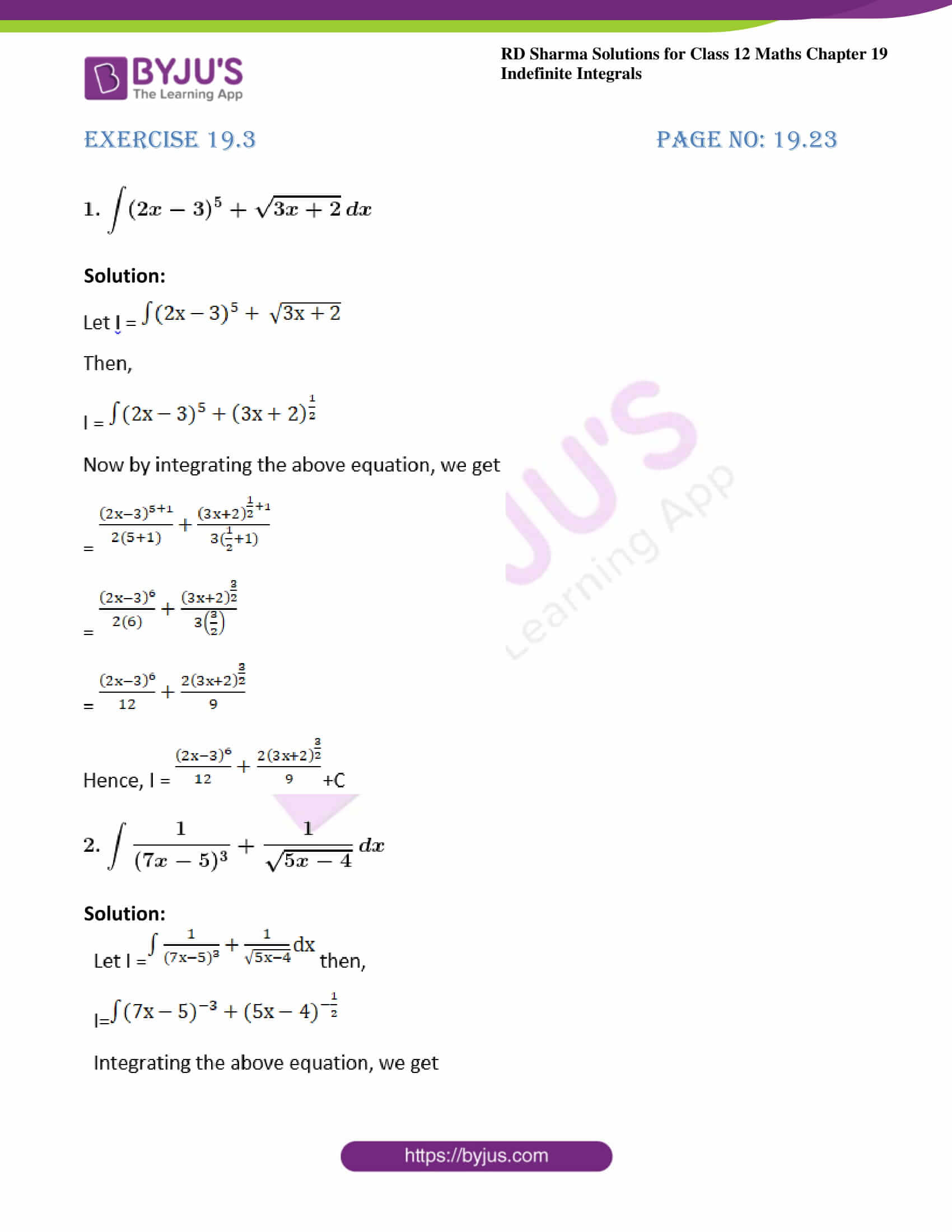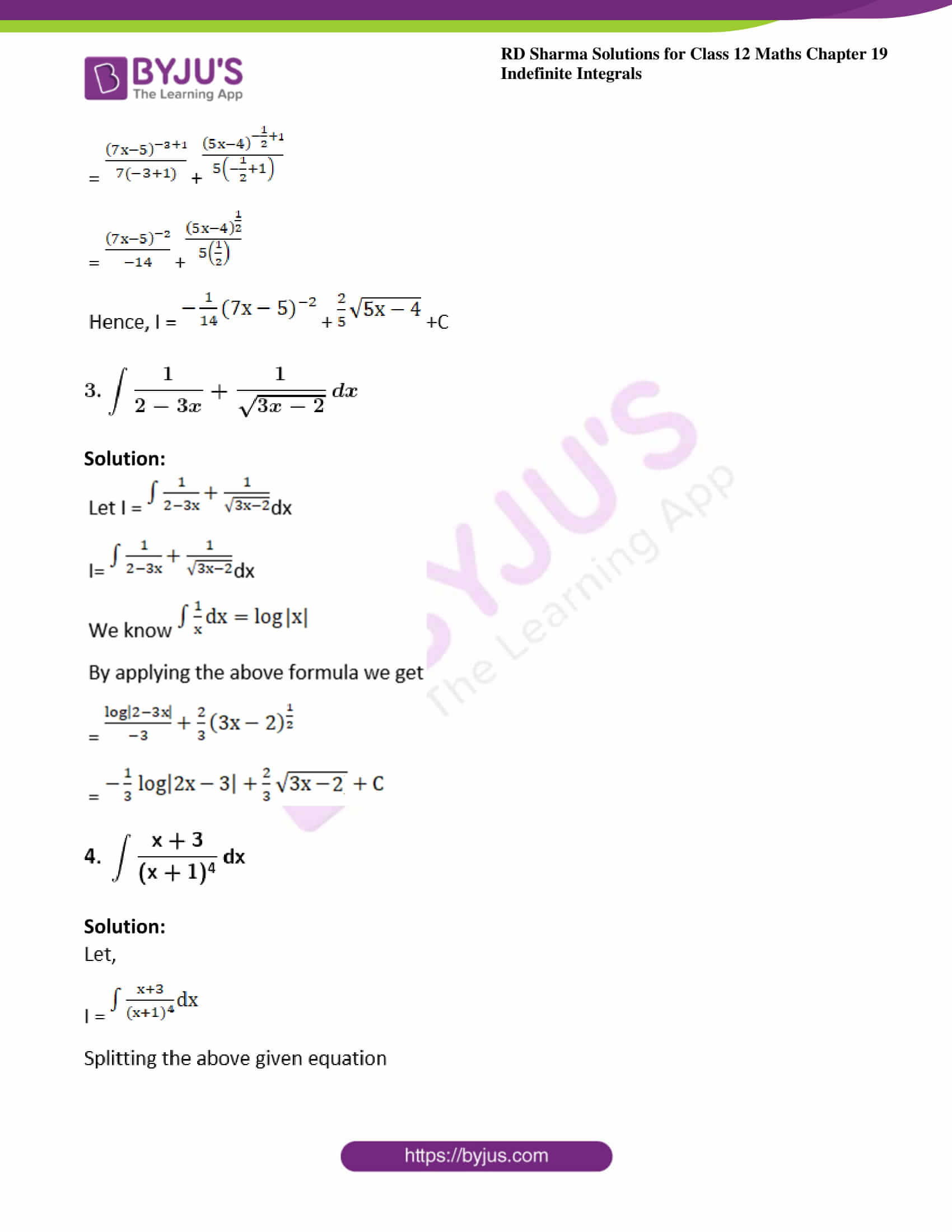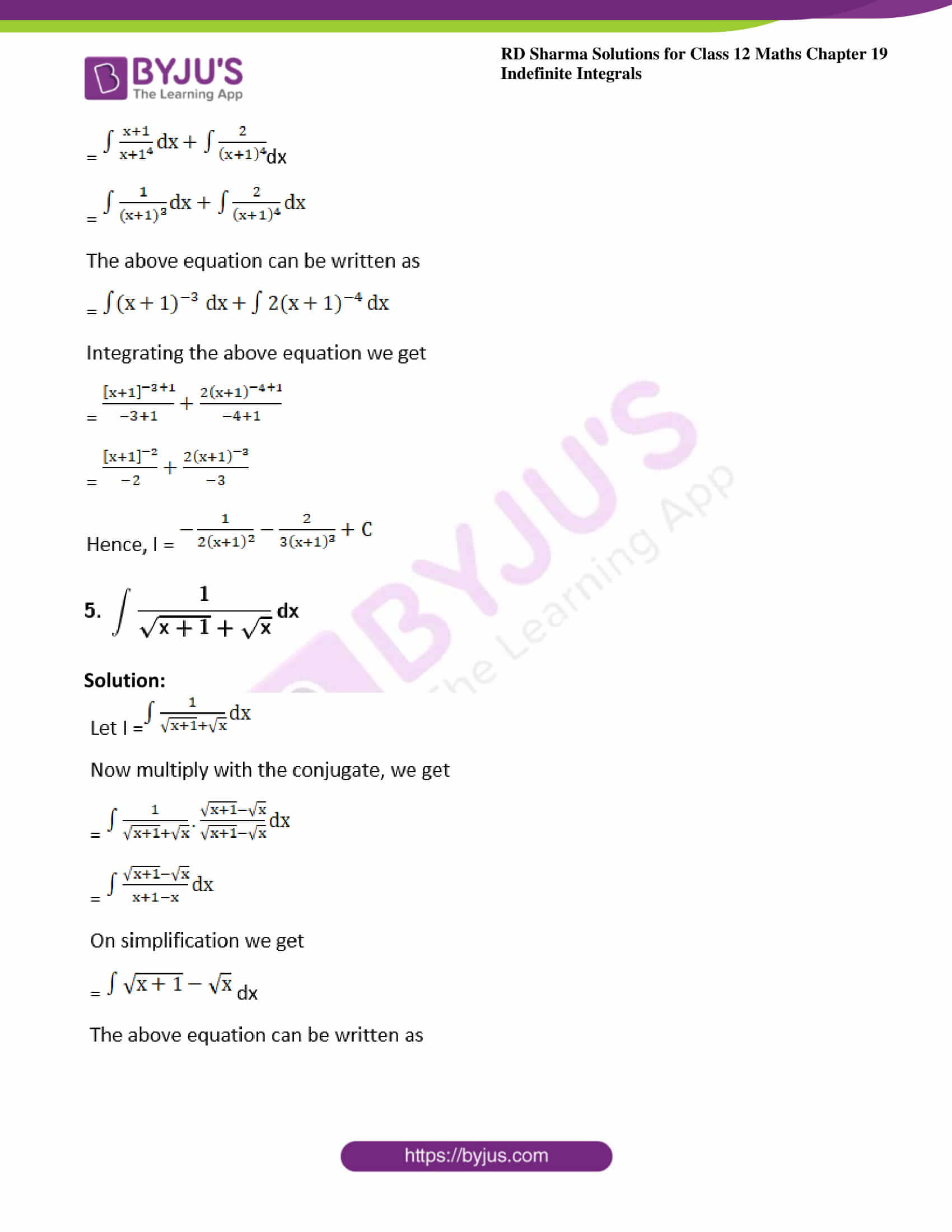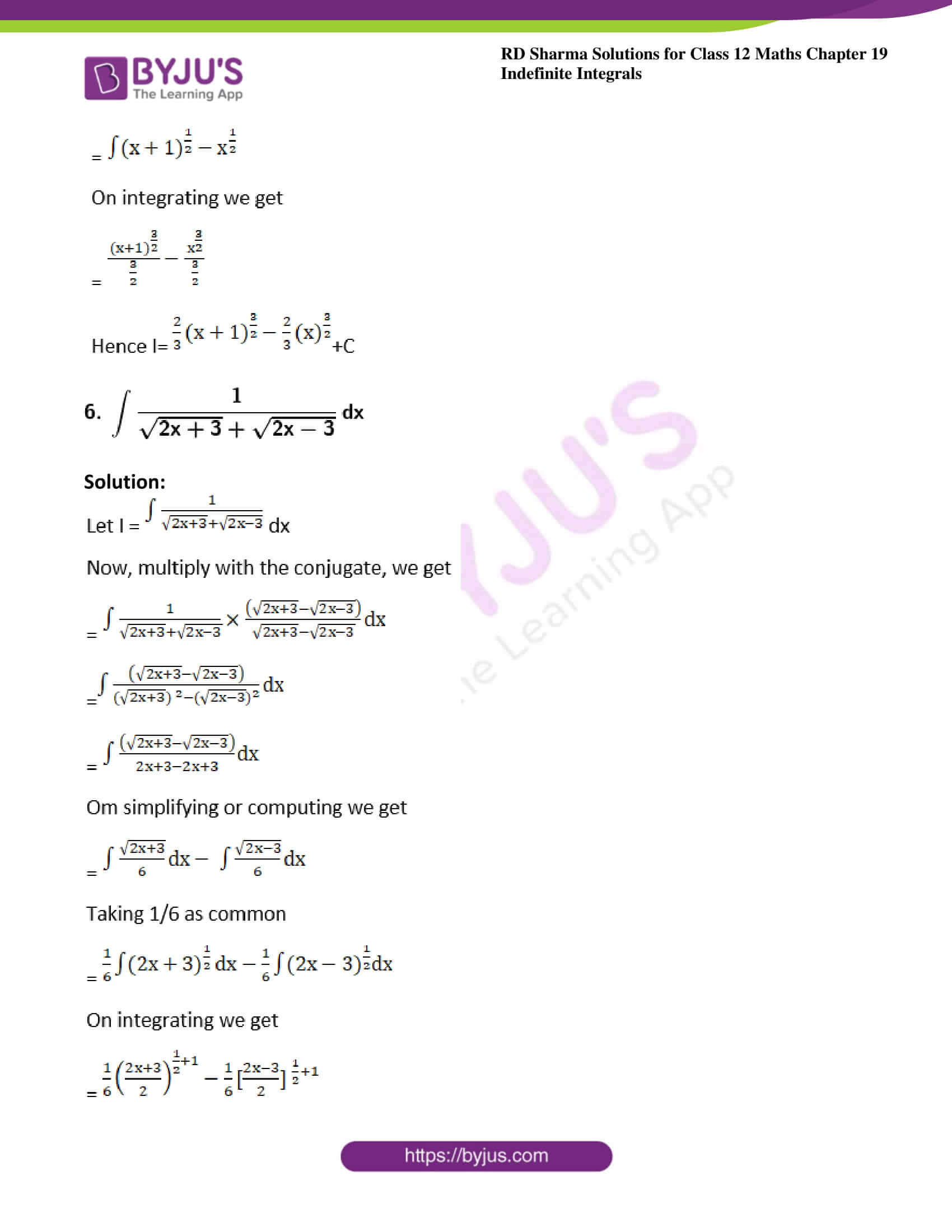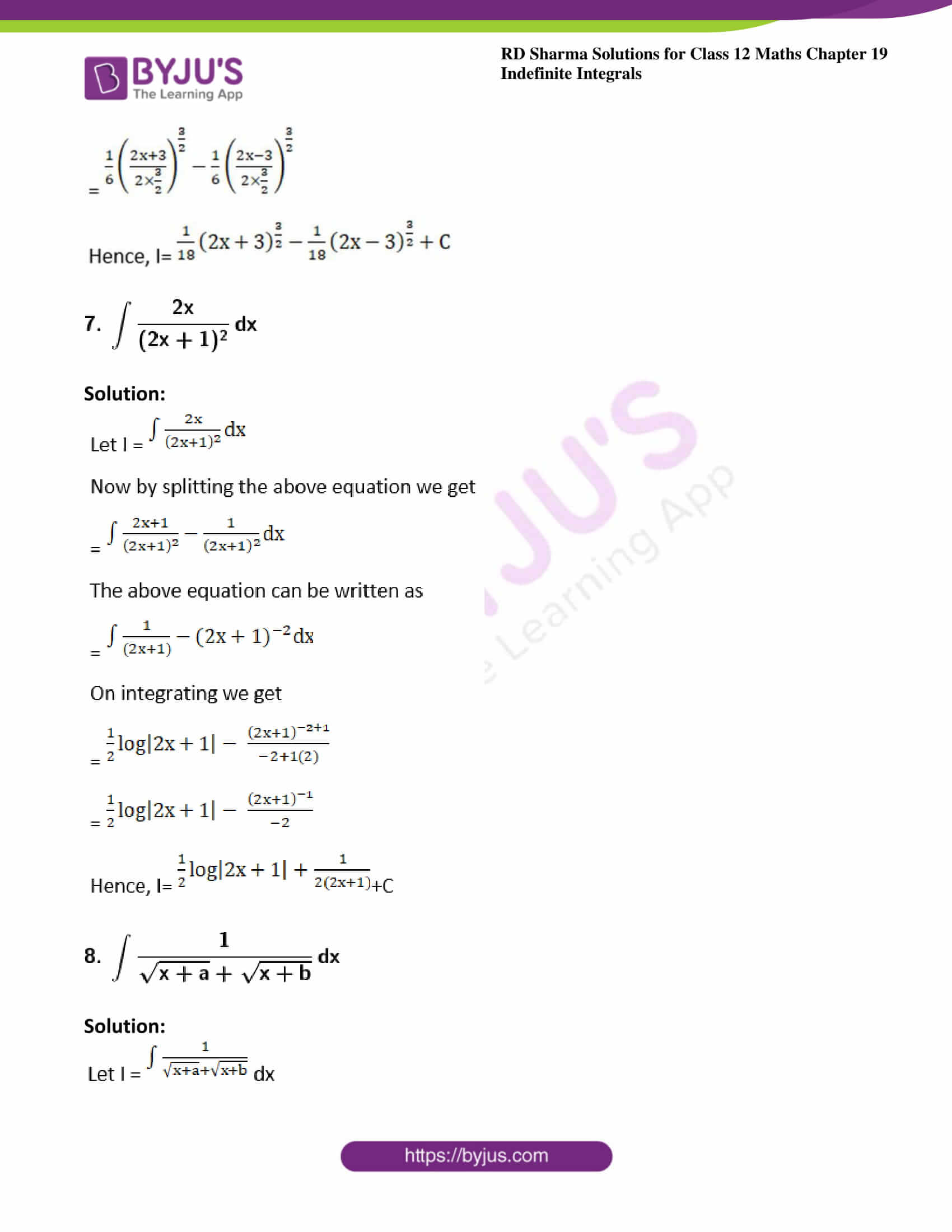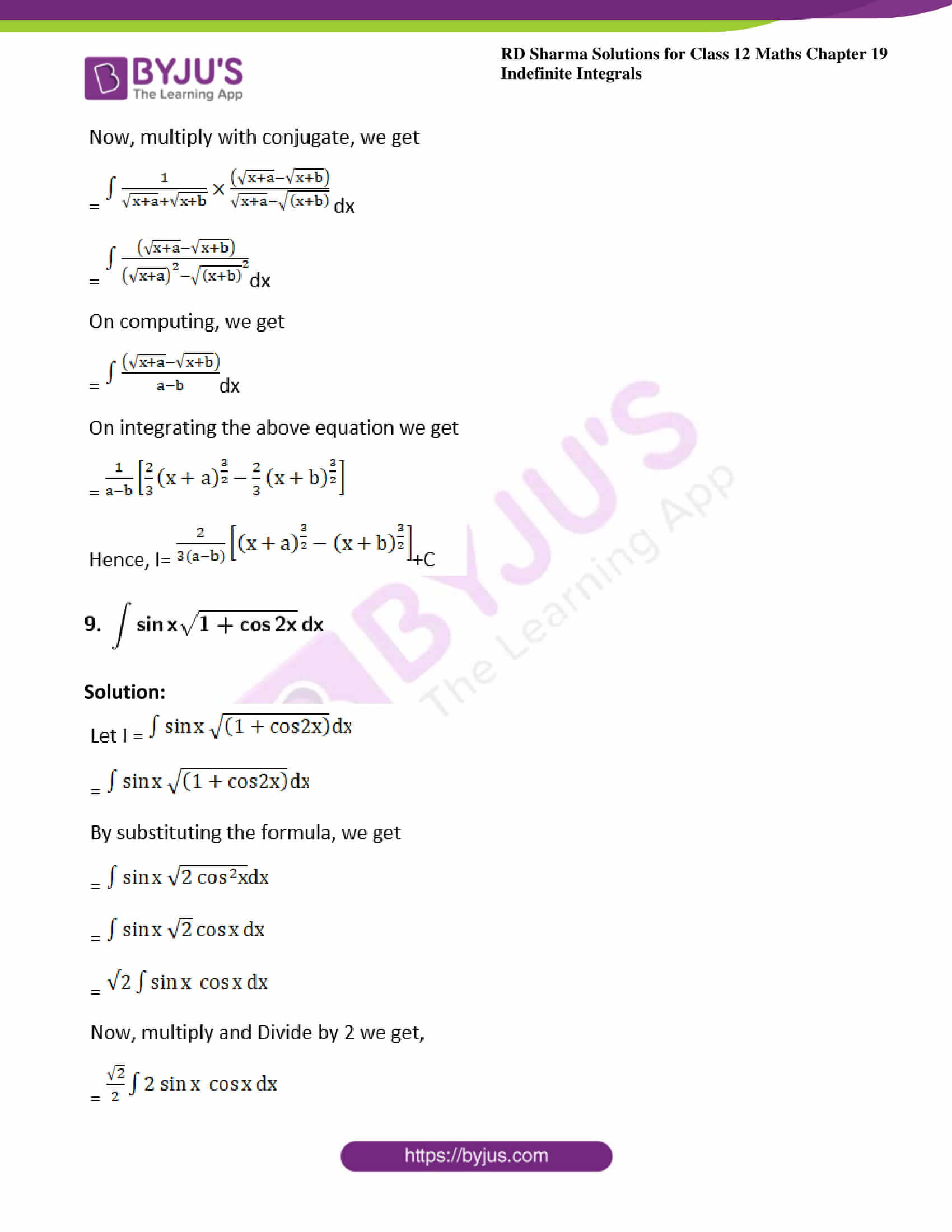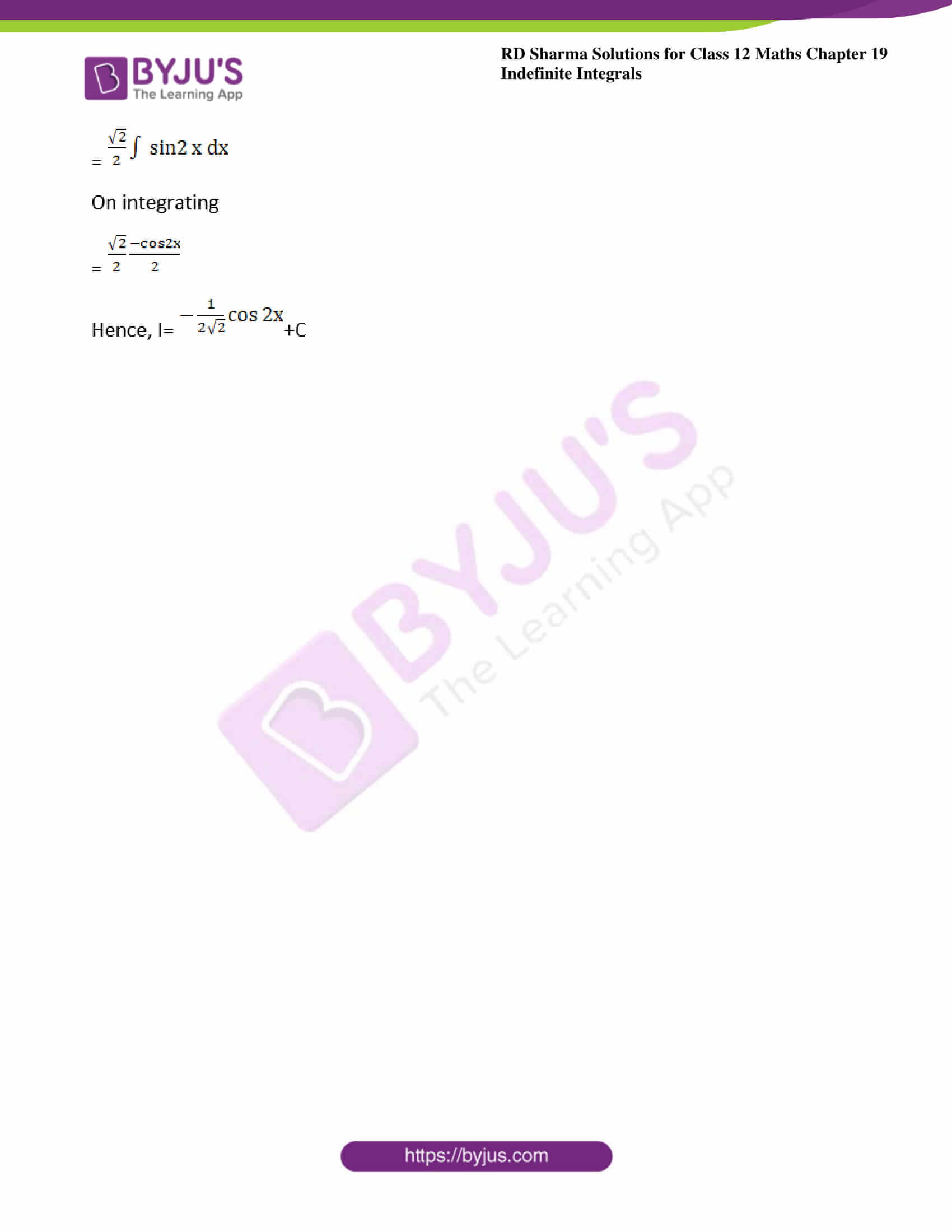### Exercise 19.3 Page No: 19.23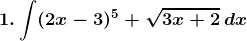Solution: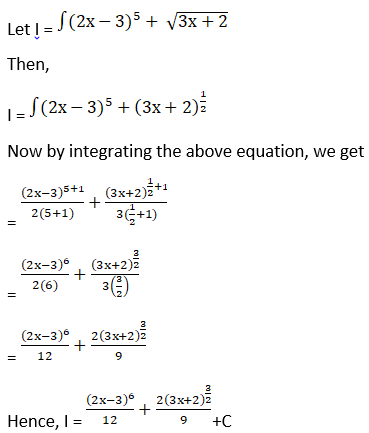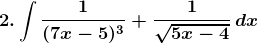Solution: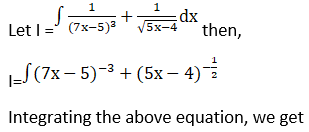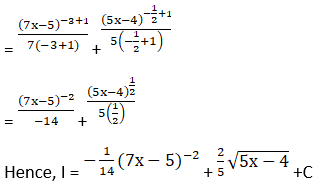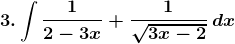Solution: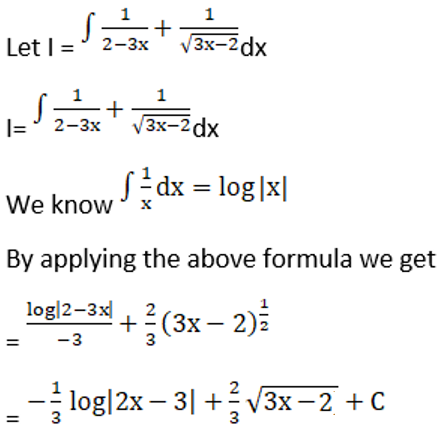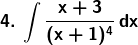Solution: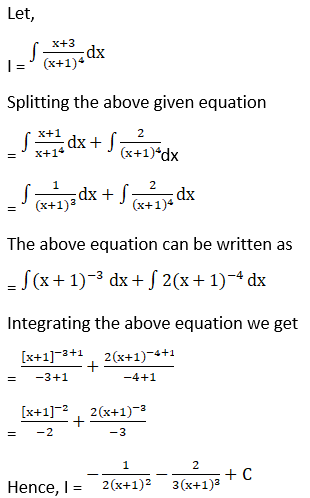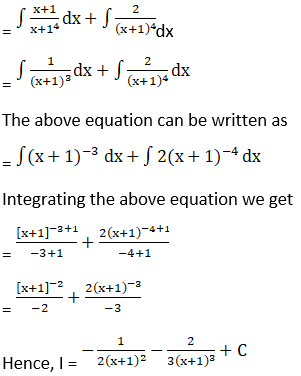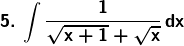Solution: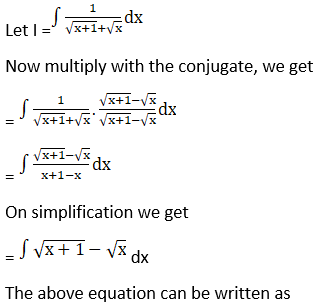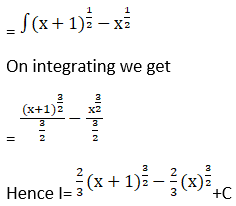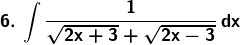Solution: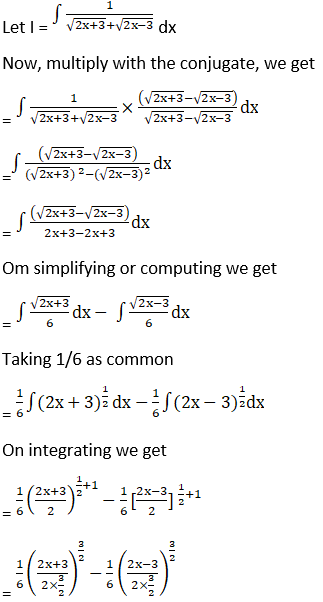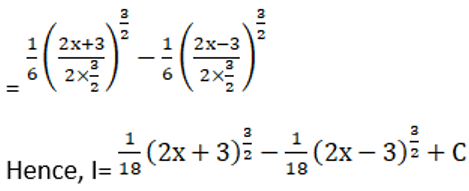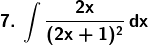Solution: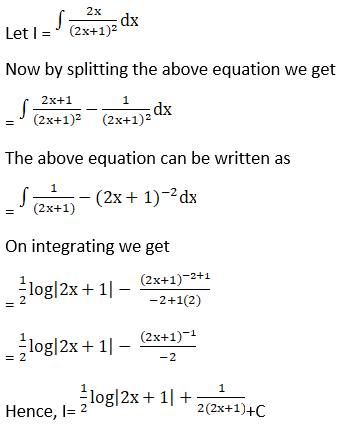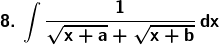Solution: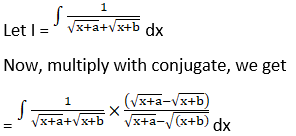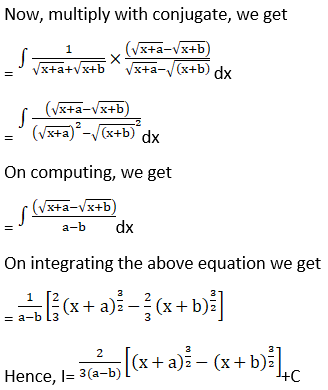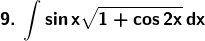Solution: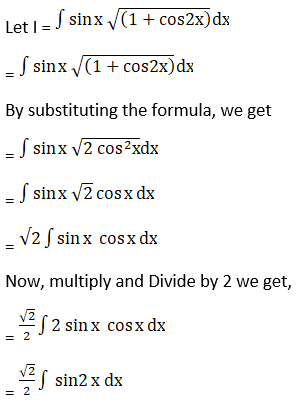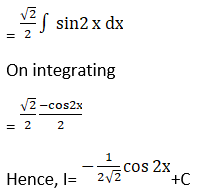### Access other exercises of RD Sharma Solutions For Class 12 Chapter 19 – Indefinite Integrals

Exercise 19.1 Solutions

Exercise 19.2 Solutions

Exercise 19.4 Solutions

Exercise 19.5 Solutions

Exercise 19.6 Solutions

Exercise 19.7 Solutions

Exercise 19.8 Solutions

Exercise 19.9 Solutions

Exercise 19.10 Solutions

Exercise 19.11 Solutions

Exercise 19.12 Solutions

Exercise 19.13 Solutions

Exercise 19.14 Solutions

Exercise 19.15 Solutions

Exercise 19.16 Solutions

Exercise 19.17 Solutions

Exercise 19.18 Solutions

Exercise 19.19 Solutions

Exercise 19.20 Solutions

Exercise 19.21 Solutions

Exercise 19.22 Solutions

Exercise 19.23 Solutions

Exercise 19.24 Solutions

Exercise 19.25 Solutions

Exercise 19.26 Solutions

Exercise 19.27 Solutions

Exercise 19.28 Solutions

Exercise 19.29 Solutions

Exercise 19.30 Solutions

Exercise 19.31 Solutions

Exercise 19.32 Solutions